# Test Prep Plan - Take a practice test

Take this practice test to check your existing knowledge of the course material. We'll review your answers and create a Test Prep Plan for you based on your results.
How Test Prep Plans work
1
2Based on your results, we'll create a customized Test Prep Plan just for you!
3Study smarter
Study more effectively: skip concepts you already know and focus on what you still need to learn.

# Quadratic Equations, Functions & Inequalities Chapter Exam

Exam Instructions:

Choose your answers to the questions and click 'Next' to see the next set of questions. You can skip questions if you would like and come back to them later with the yellow "Go To First Skipped Question" button. When you have completed the practice exam, a green submit button will appear. Click it to see your results. Good luck!

### Page 2

#### Question 7 7. Use the quadratic formula to find the solutions to the equation below.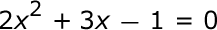#### Question 8 8. What is the b-value of the quadratic equation below?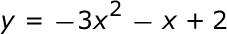### Page 3

#### Question 13 13. Which of the following are the solutions to the equation below?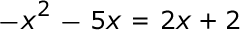#### Question 14 14. Rewrite the quadratic below in standard form: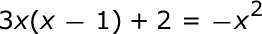#### Question 15 15. Find the roots of the equation.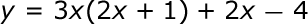### Page 5

#### Question 24 24. Which form is this quadratic in?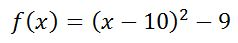#### Question 25 25. Find the solution to the equation below.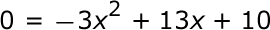### Page 6

#### Question 26 26. If the equation below told you a ball was 'h' feet in the air after 't' seconds, what would be the first step to finding out when it hit the ground?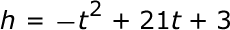#### Question 27 27. Find the solution to the equation below.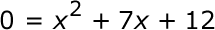#### Quadratic Equations, Functions & Inequalities Chapter Exam Instructions

Choose your answers to the questions and click 'Next' to see the next set of questions. You can skip questions if you would like and come back to them later with the yellow "Go To First Skipped Question" button. When you have completed the practice exam, a green submit button will appear. Click it to see your results. Good luck!

Support